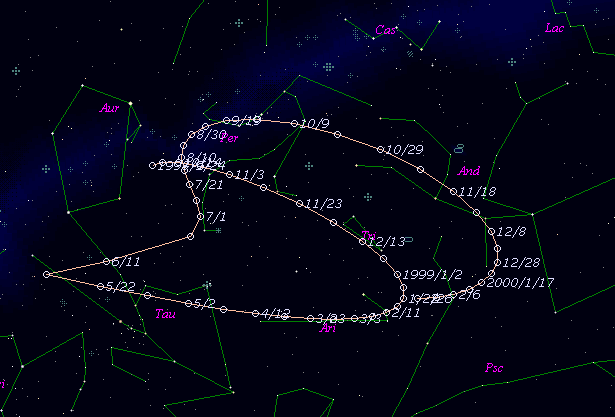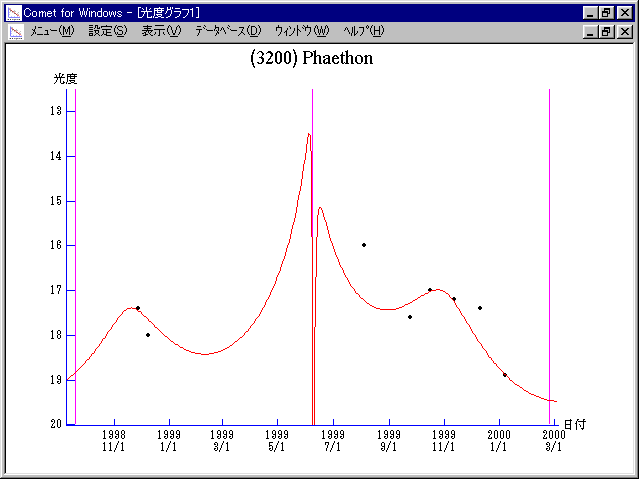\$B>.OG@1%U%!%(%H%s(B

(3200) Phaethon (1999)\$B\$B50F;MWAG(B

```   The following improved orbital elements by Kenji Muraoka, are
from 116 observations 1983 to 1996, perturbations by 9 Plantes,
Moon and 5 minor planets were taken into account.
The mean residual is +/- 0.91 arc seconds.

Epoch  =  1999 May  22.0  TT
T  =  1999 June  8.09295  TT
Peri. =  321.84748
Node  =  265.55574    (2000.0)
Incl. =   22.11465
q  =    0.1396744 AU
e  =    0.8901330
```\$B@1?^(B\$B8wEYJQ2=(B

```        H = 14.3  G = 0.15
```\$B50F;MWAG\$OB<2,7r<#;a\$N7W;;\$K\$h\$k\$b\$N\$G\$9!#(B \$B@1?^\$O(B StellaNavigator Ver.2.0 for Windows (\$B%"%9%H%m%"!<%D(B \$BJTCx(B / \$B%"%9%-!<=PHG6I4)(B) \$B\$G:n@.\$7\$?\$b\$N\$G\$9!#(B \$B8wEY%0%i%U\$O(BComet for Windows\$B\$G:n@.\$7\$?\$b\$N\$G\$9!#(B